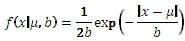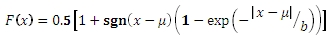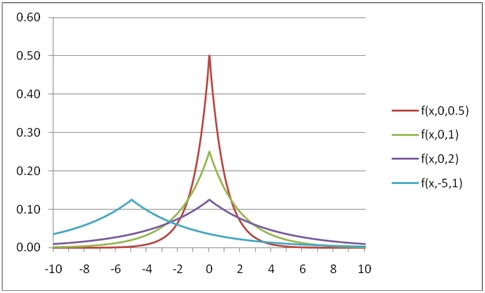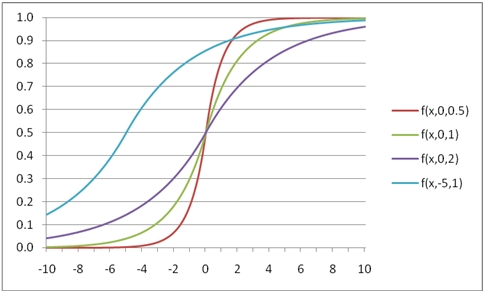# SQL Server LaPlace distribution function

LAPLACE

Updated: 31 July 2010

Use LAPLACE to calculate the probability density function or the lower cumulative distribution function of the Laplace distribution.

The formula for the probability density function is:The lower cumulative distribution function is:Syntax
SELECT [wctStatistics].[wct].[LAPLACE] (
<@X, float,>
,<@A, float,>
,<@B, float,>
,<@Cumulative, bit,>)
Arguments
@X
is the value to be evaluated. @X is an expression of type float or of a type that implicitly converts to float
@A
is the location parameter. @A is an expression of type float or of a type that implicitly converts to float
@B
is the scale parameter. @B is an expression of type float or of a type that implicitly converts to float
@Cumulative
is a logical value that determines if the probability density function ('False', 0) or the cumulative distribution function ('True', 1) is being calculated. @Cumulative is an expression of type bit or of a type that implicitly converts to bit

Return Types
float
Remarks
·         @B must be greater than zero (@B > 0).
·         The upper cumulative distribution function is equal to 1 –LAPLACE(@X,@A,@B,'True').
Examples
Calculate the probability density function:
SELECT wct.LAPLACE(0.5,0,1,'False')
This produces the following result
----------------------
0.303265329856317

(1 row(s) affected)

You can use the SeriesFloat function from the XLeratorDB/math library to generate a dataset which can be pasted into EXCEL to generate a graph of the probability density function.
SELECT SeriesValue
,wct.LAPLACE(SeriesValue, 0, 1, 'False') as [f(x,0,0.5)]
,wct.LAPLACE(SeriesValue, 0, 2, 'False') as [f(x,0,1)]
,wct.LAPLACE(SeriesValue, 0, 4, 'False') as [f(x,0,2)]
,wct.LAPLACE(SeriesValue, -5, 4, 'False') as [f(x,-5,1)]
FROM wct.SeriesFloat(-10, 10, .1,NULL,NULL)
This is an EXCEL-generated graph of the resultsCalculate the lower cumulative distribution function:
SELECT wct.LAPLACE(0.5,0,1,'True')
This produces the following result
----------------------
0.696734670143683

(1 row(s) affected)

You can use the SeriesFloat function from the XLeratorDB/math library to generate a dataset which can be pasted into EXCEL to generate a graph of the cumulative distribution function.
SELECT SeriesValue
,wct.LAPLACE(SeriesValue, 0, 1, 'True') as [f(x,0,0.5)]
,wct.LAPLACE(SeriesValue, 0, 2, 'True') as [f(x,0,1)]
,wct.LAPLACE(SeriesValue, 0, 4, 'True') as [f(x,0,2)]
,wct.LAPLACE(SeriesValue, -5, 4, 'True') as [f(x,-5,1)]
FROM wct.SeriesFloat(-10, 10, .1,NULL,NULL)
This is an EXCEL-generated graph of the resultsCalculate the upper cumulative distribution function:
SELECT 1 - wct.LAPLACE(0.5,0,1,'True')
This produces the following result
----------------------
0.303265329856317

(1 row(s) affected)

### SupportCopyright 2008-2023 Westclintech LLC         Privacy Policy        Terms of Service